# System of equations - high school - math problems

#### Number of problems found: 371

• Two equationsSolve equations (use adding and subtracting of linear equations): -4x+11y=5 6x-11y=-5
• Linear systemSolve a set of two equations of two unknowns: 1.5x+1.2y=0.6 0.8x-0.2y=2
• Non linear eqsSolve the system of non-linear equations: 3x2-3x-y=-2 -6x2-x-y=-7
• Three unknownsSolve the system of linear equations with three unknowns: A + B + C = 14 B - A - C = 4 2A - B + C = 0
• Elimination methodSolve system of linear equations by elimination method: 5/2x + 3/5y= 4/15 1/2x + 2/5y= 2/15
• Linsys2Solve two equations with two unknowns: 400x+120y=147.2 350x+200y=144
• Touch x-axisFind the equations of circles that pass through points A (-2; 4) and B (0; 2) and touch the x-axis.
• A candleA candle shop sells scented candles for \$16 each and unscented candles for \$10 each. The shop sells 28 candles today and makes \$400. a. Write a system of linear equations that represents the situation. b. Solve the system to answer the questions: How many
• Null points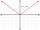Calculate the roots of the equation: ?
• There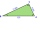There is a triangle ABC: A (-2,3), B (4, -1), C (2,5). Determine the general equations of the lines on which they lie: a) AB side, b) height to side c, c) Axis of the AB side, d) median ta to side a
• Divide 8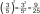Divide 6840 by x y and z, in such a way that x has twice as much as y, who has half as much as z
• AP - basicsDetermine first member and differentiate of the the following sequence: a3-a5=24 a4-2a5=61
• Together m+wWomen 30%. Men are 360 more. How many are together?
• Inverse matrix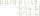Find out inverse by Gauss elimination or by reduction method. A=[2/3. 1 -3. 1/3]
• Find d 2Find d in an A. P. whose 5th term is 18 and 39th term is 120.
• AP 6Calculate the first five items of an arithmetic sequence if it is given: a2 – a3 + a5 = 20 a1 + a6 = 38
• Vector perpendicularFind the vector a = (2, y, z) so that a⊥ b and a ⊥ c where b = (-1, 4, 2) and c = (3, -3, -1)
• Intersections 3Find the intersections of the circles x2 + y2 + 6 x - 10 y + 9 = 0 and x2 + y2 + 18 x + 4 y + 21 = 0
• LegsCancer has 5 pairs of legs. The insect has 6 legs. 60 animals have a total of 500 legs. How much more are cancers than insects?
• Three numbers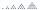What is three numbers which have the property: the sum of the reciprocals of the first and second numbers is 12/7, the first and third 11/24 and the second and the third 3/8.

Do you have an interesting mathematical word problem that you can't solve it? Submit a math problem, and we can try to solve it.

We will send a solution to your e-mail address. Solved examples are also published here. Please enter the e-mail correctly and check whether you don't have a full mailbox.

Please do not submit problems from current active competitions such as Mathematical Olympiad, correspondence seminars etc...

Do you have a system of equations and looking for calculator system of linear equations? System of equations - math word problems. Examples for secondary school students.